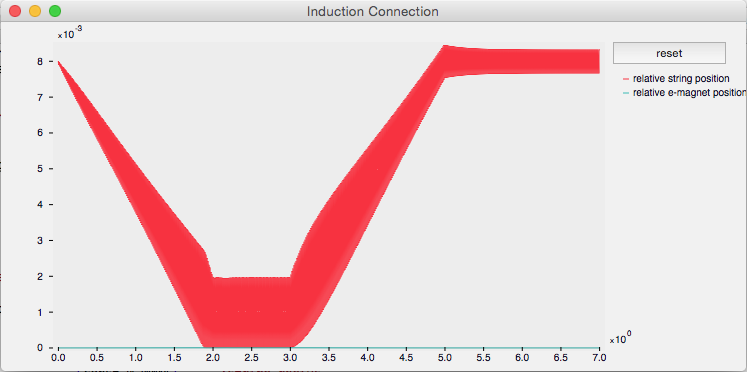# Phase 2-Induction

## The Induction Connection: The Creation of a Physical Model of the Interaction Between an Electromagnet and Vibrating Body

Once Joël had derived a working algorithm for induction, the new Induction Connection was added to the Modalys codebase.

The documentation for the Induction Connection is available through the Modalys documentation pages. A summary follows:

The connection requires that two objects be created, each with an access. The induction connection specifies the parameters of the interaction between the two accesses. One of the objects acts as the electromagnet, the other as the resonating body. We refer to these as the “electromagnet” (no surprise there) and the “string” for convenience, but either object can be anything (wood, a column of air, etc.).

The connection further requires the presence of an input signal. This signal is equivalent to the current introduced into the electromagnet, which controls the polarity of the resulting magnetic field, and thus the force applied to the resonating object.

A number of physical parameters must be set, including the gap between the two objects, the length and radius of the electromagnet object, and the radius and permeability of the string object. These parameters are used only to compute the force of the interaction, they have nothing to do with the physical characteristics of the objects themselves. As such they are set when creating the induction connection, not when creating the objects to which they apply

The gap size is inversely related to the strength of the interaction. Just as in the real-world model, if the gap is too small the resonating object will strike the electromagnet. In order to mimic this potential physical contact, there is a strike connection invoked when the gap reaches zero. This is of course physically accurate, though not necessarily required in the virtual world of Modalys. However the presence of this strike connection, which makes a gap of zero impossible, also avoids the divide-by-zero error that would otherwise result.

The ‘induction connection can be created using the following Lisp syntax (the default values are shown for each of the physical parameters):

```(make-connection 'induction string-access electromagnet-access signal-controller gap
(const 0.02) ;electromagnet length
(const 8.737434e-4) ;string permeability
```

There are a couple of quirks to the implementation as it stands:

• The given default parameters create a relatively weak interaction force. More interesting (and certainly more audible) results can be obtained by either significantly boosting the input signal, or raising the string permeability.
• The two objects are by default floating freely in space. When the magnet exerts a force on the string, they will both move closer together if they are not specifically secured in place.
• The frequency of the vibration string is twice that of the input frequency.

### Introductory Example

This first example demonstrates basic induction on a simple string. An electromagnet and a string are created, and a sine is used as the input signal. The electromagnet begins 8 mm from the string (gap = 0.008 meters), moves to 1 mm, at which point the two objects strike each other, then moves back to 8 mm.Graph of the positions of the string (blue) and electromagnet (red)

```
;;;-*-Mode: Lisp; Package: MODALYS -*-

;;;----------------------------------------------------------------------
;;; Modalys, Induction.
;;; This demonstrates the "induction" connection to simulate the action
;;; of an electromagnet over a metallic string.
;;;----------------------------------------------------------------------

(new)
(set-message-level 3)

;;; our piano string with an access on it:

(setq string (make-object 'mono-string))

(set-pitch string 'tension 110)
(setq string-access (make-access string (const .75) 'trans0))

;;; the electromagnet, modeled as a simple harmonic oscillator, with an access on it:

(setq electromagnet (make-object 'mono-two-mass
(small-mass 0.1)
(large-mass .1)
(stiffness0 5000)
(freq-loss0 100)
(const-loss0 50)))

(setq electromagnet-access (make-access electromagnet (const 1) 'trans0))

;;; make the electromagnet steady over time
;;; (on the graph, it will be the flat, unmoving line at the bottom)

(setq electromagnet-handle (make-access electromagnet (const 0) 'trans0))
(make-connection 'position electromagnet-handle (const 0))

;;; input sound (sine wave):

(setq factor (make-controller 'constant 1 50))
; this allows us to boost the input signal as much as necessary to get a reasonable output
(setq raw-sine (make-controller 'sine 1 110 45))
(setq sine-controller (make-controller 'arithmetic 1 "*" raw-sine factor))

;;; variable gap between the electromagnet and the string
;;; We are forcing the electromagnet towards the string so they collide, and then moving it away

(setq gap (make-controller 'envelope 1
(list  (list 0.0   0.008)
(list 2   0.001)
(list 3   0.001) ; 1 second worth of collision
(list 5   0.008))))

;;; the induction connection per se

(make-connection 'induction string-access electromagnet-access sine-controller gap
(const 0.02)        ;electromagnet length
(const 8.737434e-4) ;string permeability
)
;;; otherwise default values are built-in:
;;; (make-connection 'induction string-access electromagnet-access sine-controller gap)

;;; make listening point on string. How does this sound?

(setq string-out (make-access string (const .3) 'trans0))
(make-point-output string-out)

;;; let us plot the position at the string's access

(setq pos1 (make-controller 'access-position 1 string-access))
(setq pos2 (make-controller 'access-position 1 electromagnet-access))
(setq force (make-controller 'access-force 1 electromagnet-access))
(setq graph (make-plot))
(plot-value graph "relative string position" pos1)
(plot-value graph "relative e-magnet position" pos2)
;;(plot-value graph "electromagnet force" force)

;;; run the synthesis, plot the position, and play the sound

(run 7)
(plot graph "Induction Connection")
(play)
```

For more examples, continue on to Induction Examples 1